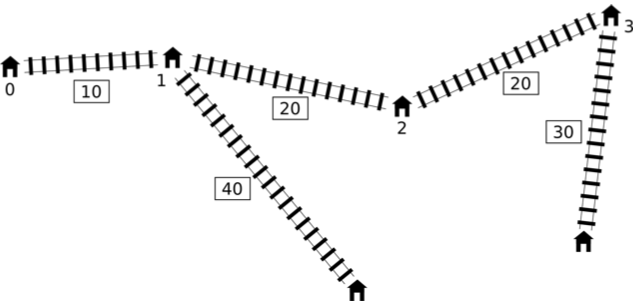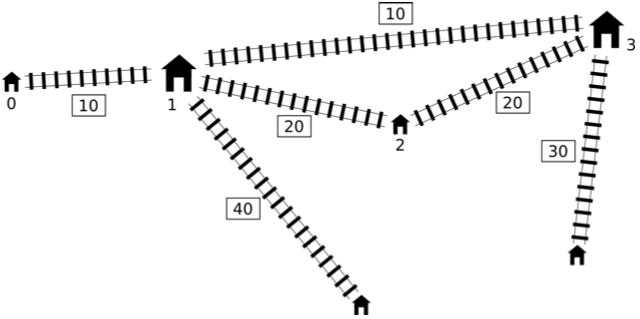# #237. 【IOI2016】shortcut

Pavel 有一个非常简单的铁路玩具。 它有一条含有 $n$ 个车站的主干线并且连续编号为 $0$ 到 $n−1$。车站 $0$ 和车站 $n−1$ 就在这条主干线的两端。其中车站 $i$ 和车站 $i+1$ 之间的距离为 $l_i$ 厘米（$0\le i < n - 1$）。Pavel 现正规划一条快捷方式：一条在主干线中两个不相同的车站之间（它们可能相邻）的快速干线。这条快速干线无论是连接哪两个车站，它的长度都将会恰好是 $c$ 厘米。

Pavel 就是想建造一条快速干线，使得有了这条快速干线后新的铁路网络的直径能达到最小值。

### 实现细节

• long long find_shortcut(int n, std::vector<int> l, std::vector<int> d, int c)
• n：主干线中的车站数目，
• l：主干线中车站之间的距离（数组的长度为 $n - 1$），
• d：支线的长度（数组的长度为 $n$），
• c：新快速干线的长度。
• 函数应该返回加入新快速干线后铁路网络直径的最小可能值。

### 样例一

find_shortcut(4, [10, 20, 20], [0, 40, 0, 30], 10)### 样例二

find_shortcut(9, [10, 10, 10, 10, 10, 10, 10, 10], [20, 0, 30, 0, 0, 40, 0, 40, 0], 30)

### 样例三

find_shortcut(4, [2, 2, 2], [1, 10, 10, 1], 1)

### 样例四

find_shortcut(3, [1, 1], [1, 1, 1], 3)

### 子任务

19$10$
214$100$
38$250$
47$500$
533$3000$
622$100000$
74$300000$
83$1000000$

### 评测方式

• 第一行：两个整数 $n$ 和 $c$，
• 第二行：整数 $l_0, l_1, \ldots, l_{n - 2}$，
• 第三行：整数 $d_0, d_1, \ldots, d_{n - 1}$。